• 自适应滤波器自适应滤波器 自适应滤波器 以是连续域的或是离散域的。离散域自适应滤波器由一组抽头延迟线、可变加权系数和自动调整系数的机构组成。附图表示一个离散域自适应滤波器用于模拟未知离散系统的信号流...
• 河南科技学院新科学院 2014届本科毕业论文设计 基于matlab自适应滤波器滤波器的设计及实现 学生姓名 倪 闯 所在院系新科学院电气工程系 所学专业电气工程及其自动化 导师姓名 孔晓红 完成时间2014年4月10日 基于...
• 自适应滤波器自适应滤波器自适应滤波器自适应自适应滤波器滤波器自适应滤波器
• 1. 自适应滤波器简介   在很多信号处理系统中，并没有信号的先验统计特性，不能使用某一固定参数的滤波器来处理，比如信道均衡、回声消除以及其他因素之间的系统模型等，均采用了调整系数的滤波器，称为自适应...
1. 自适应滤波器简介
在很多信号处理系统中，并没有信号的先验统计特性，不能使用某一固定参数的滤波器来处理，比如信道均衡、回声消除以及其他因素之间的系统模型等，均采用了调整系数的滤波器，称为自适应滤波器。这样的滤波器结合了允许滤波器系数适应于信号统计特性的算法。

自适应滤波器的特点

没有关于待提取信息的先验统计知识
直接利用观测数据依据某种判据在观测过程中不断递归更新
最优化

自适应滤波器分类

按结构分：横向结构、格型结构
按算法分：随机梯度、最小二乘
按处理方式分：成批处理、递归处理

自适应滤波器应用

噪声抵消
回音消除
谱线增强
通道均衡
系统辨识

2. 自适应滤波器原理
2.1 原理概述
自适应滤波器的原理框图如下图所示，输入信号x(n) 通过参数可调数字滤波器后产生输出信号 y(n)，将其与***期望信号d(n)*进行比较，形成误差信号e(n)， 通过自适应算法对滤波器参数进行调整，最终使 *e(n)*的均方值最小。自适应滤波可以利用前一时刻已得的滤波器参数的结果，自动调节当前时刻的滤波器参数，以适应信号和噪声未知的或随时间变化的统计特性，从而实现最优滤波。自适应滤波器实质上就是一种能调节自身传输特性以达到最优的维纳滤波器。自适应滤波器不需要关于输入信号的先验知识，计算量小，特别适用于实时处理。维纳滤波器参数是固定的，适合于平稳随机信号。卡尔曼滤波器参数是时变的，适合于非平稳随机信号。然而，只有在信号和噪声的统计特性先验已知的情况下，这两种滤波技术才能获得最优滤波。在实际应用中，常常无法得到信号和噪声统计特性的先验知识。在这种情况下，自适应滤波技术能够获得极佳的滤波性能，因而具有很好的应用价值。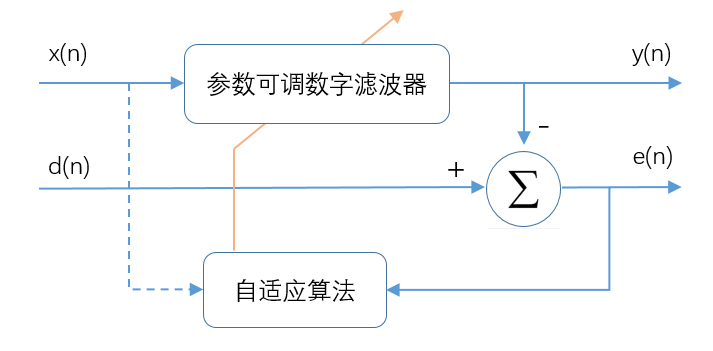2.2 模型分析
自适应滤波器的详细模型如下图所示。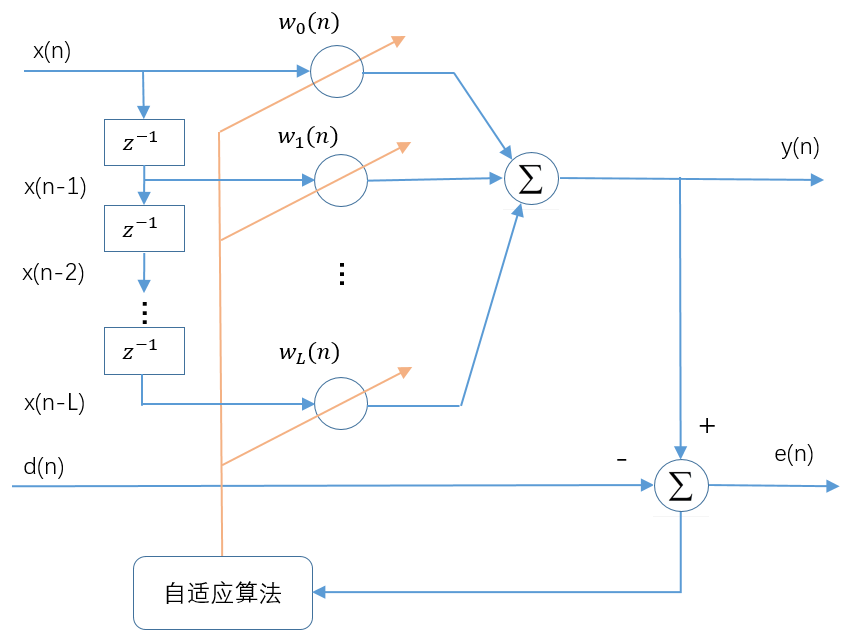输入信号矢量：
$\boldsymbol{x}(n)=[x(n) x(n-1) \ldots x(n-L)]^{T}$
输出$y(n)$为：
$y(n)=\sum_{k=0}^{L} w_{k}(n) x(n-k)$
自适应线性组合器的L+1个权系数构成一个权系数矢量，称为权矢量，用$w(n)$表示，即
$\boldsymbol{w}(n)=\left[w_{0}(n) w_{1}(n) \ldots w_{L}(n)\right]^{T}$
因此$y(n)$可以表示为：
$y(n)=x^{T}(n) \boldsymbol{w}(n)=\boldsymbol{w}^{T}(n) \boldsymbol{x}(n)$
误差信号为：
$e(n)=d(n)-y(n)=d(n)-x^{T}(n) w(n)=d(n)-\boldsymbol{w}^{T}(n) \boldsymbol{x}(n)$
自适应线性组合器按照误差信号均方值最小的准则，即：
$\xi(n)=E\left[e^{2}(n)\right]=E\left[d^{2}(n)\right]+\boldsymbol{w}^{T}(n) E\left[\boldsymbol{x}(n) \boldsymbol{x}^{T}(n)\right] \boldsymbol{w}(n)-2 E\left[d(n) \boldsymbol{x}^{T}(n)\right] \boldsymbol{w}(n)$
输入信号的自相关矩阵为：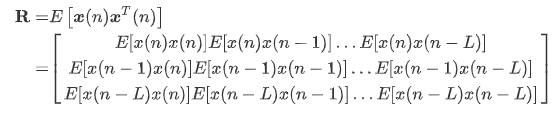期望信号与输入信号的互相关矩阵为：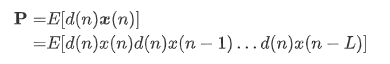则均方误差的简单表示形式为：
$\xi(n)=E\left[d^{2}(n)\right]+\boldsymbol{w}^{T} R \boldsymbol{w}-2 P^{T} \boldsymbol{w}$
从该式可看出，在输入信号和参考响应都是平稳随机信号的前提下，均方误差是权矢量的各分量的二次函数。该函数图形是L+2维空间中一个中间下凹的超抛物面，有唯一的最低点,该曲面称为均方误差性能曲面，简称性能曲面。
均方误差性能曲面的梯度：
$\nabla=\frac{\partial \xi}{\partial w}=2 \boldsymbol{R} \boldsymbol{w}-2 \boldsymbol{P}$
令梯度 $\nabla$ 等于零，可求得最小均方误差对应的最佳权矢量或维纳解 $\boldsymbol{W}^{*}$，解得 $\boldsymbol{w}^{*}=\boldsymbol{R}^{-1} \boldsymbol{P}$。
虽然维纳解的表达式我们知道了，但仍然有几个问题：

需要知道$R$和$P$，而这两个都是我们事先不知道的
矩阵的逆需要的计算量太大：$O(n^3)$
如果信号是非平稳的，$R$和$P$每次都不一样，需要重复计算

2.3 梯度下降法
一般情况下，我们使用递归的方法来寻找多变量函数的最小值，其性能指标就是MSE(Mean Square Error)，它是滤波器系数的二次函数，因此该函数具有唯一的最小值。一般是采用梯度下降的方法来进行迭代搜索出最小值，梯度下降又分为梯度下降、随机梯度下降和批量梯度下降。
使用迭代搜索的方式一般都只能逼近维纳解，并不等同于维纳解。
下面我们先来看梯度下降法，再来看下前面的公式：（梯度下降的原理可参考我的另一篇文章：梯度下降算法 线性回归拟合）
误差信号为：
$e(n)=d(n)-y(n)=d(n)-x^{T}(n) w(n)=d(n)-\boldsymbol{w}^{T}(n) \boldsymbol{x}(n)$
均方误差为：
$J=E\left[e^{2}(n)\right]=E\left[d^{2}(n)\right]+\boldsymbol{w}^{T}(n) E\left[\boldsymbol{x}(n) \boldsymbol{x}^{T}(n)\right] \boldsymbol{w}(n)-2 E\left[d(n) \boldsymbol{x}^{T}(n)\right] \boldsymbol{w}(n)$
利用最陡下降算法，沿着性能曲面最速下降方向（负梯度方向）调整滤波器强权向量，搜索性能曲面的最小点，计算权向量的迭代公式为：
$\boldsymbol{w}(n+1)=\boldsymbol{w}(n)+\mu(-\nabla \boldsymbol{J})$
其中$u$为步长因子，$u$的取值需要满足下式，其中$\lambda_{max}$表示输入信号自相关矩阵的最大特征值。
$\left|1-2 \mu \lambda_{\max }\right|<1 \Rightarrow 0<\mu<\frac{1}{\lambda_{\max }}$
由于计算特征值比较复杂，有时为了避免计算特征值，可采用计算矩阵迹的方法，因为自相关矩阵$\mathbf{R}$是正定的，因此有：
$t_{r}[\mathbf{R}]=\sum_{k=0}^{L} \lambda_{k}>\lambda_{\max }$
这里，$t_{r}[\mathbf{R}]$是$\mathbf{R}$的迹，它可以用输入信号的取样值进行估计，即：
$t_{r}[\mathbf{R}]=\sum_{k=0}^{L} E\left[x_{i}^{2}(n)\right]$
因此有：
$0<\mu
在梯度下降算法中，为获得系统的最佳维纳解，需要知道输入信号和期望信号的相关信息，当期望信号未知时，就无法确定它们的相关特性，必须对梯度向量进行估计。
LMS自适应算法直接利用瞬态均方误差对瞬时抽头向量（滤波器系数）求梯度：
$\hat{\nabla} \boldsymbol{J}=\frac{\partial\left(e^{2}(n)\right)}{\partial w(n)}=\left[2 e(n) \frac{\partial e(n)}{\partial w_{1}(n)}, 2 e(n) \frac{\partial e(n)}{\partial w_{2}(n)}, \cdots, 2 e(n) \frac{\partial e(n)}{\partial w_{M}(n)}\right]^{T}=-2 e(n) \boldsymbol{x}(n)$
由此可得传统LMS自适应滤波算法流程如下：
KaTeX parse error: Unknown column alignment: y at position 16:
\begin{array}
y̲y(n)=\boldsymbo…
下面我们来看一个实际的仿真：
close all;clear all;clc;

%% 产生测试信号
fs = 1;
f0 = 0.02;
n = 1000;
t = (0:n-1)'/fs;
xs = cos(2*pi*f0*t);
ws = awgn(xs, 30, 'measured');

% figure;
% subplot(211);plot(t, xs);title('原始信号');
% subplot(212);plot(t, ws);title('加噪信号');

M  = 20 ;   % 滤波器的阶数
xn = ws;
dn = xs;
% rho_max = max(eig(ws*ws.'));   % 输入信号相关矩阵的最大特征值
% mu = (1/rho_max) ;    % 收敛因子 0 < mu < 1/rho
mu = 0.001;
[yn,W,en] = lmsFunc(xn,dn,M,mu);

figure;
ax1 = subplot(211);
plot(t,ws);grid on;ylabel('幅值');xlabel('时间');
ylim([-1.5 1.5]);title('LMS滤波器输入信号');

ax2 = subplot(212);
plot(t,yn);grid on;ylabel('幅值');xlabel('时间');title('LMS滤波器输出信号');

figure;plot(en);grid on;title('误差');

其中LMS滤波器函数如下：
% 输入参数:
%   xn   输入的信号
%   dn   所期望的响应
%   M    滤波器的阶数
%   mu   收敛因子(步长)
% 输出参数:
%   W    滤波器系数矩阵
%   en   误差序列
%   yn   滤波器输出
function [yn, W, en]=lmsFunc(xn, dn, M, mu)
itr = length(xn);
en = zeros(itr,1);
W  = zeros(M,itr);    % 每一列代表-次迭代,初始为0
% 迭代计算
for k = M:itr                  % 第k次迭代
x = xn(k:-1:k-M+1);        % 滤波器M个抽头的输入
y = W(:,k-1).' * x;        % 滤波器的输出
en(k) = dn(k) - y ;        % 第k次迭代的误差
% 滤波器权值计算的迭代式
W(:,k) = W(:,k-1) + 2*mu*en(k)*x;
end

yn = inf * ones(size(xn)); % 初值为无穷大是绘图使用，无穷大处不会绘图
for k = M:length(xn)
x = xn(k:-1:k-M+1);
yn(k) = W(:,end).'* x;  % 最终输出结果
end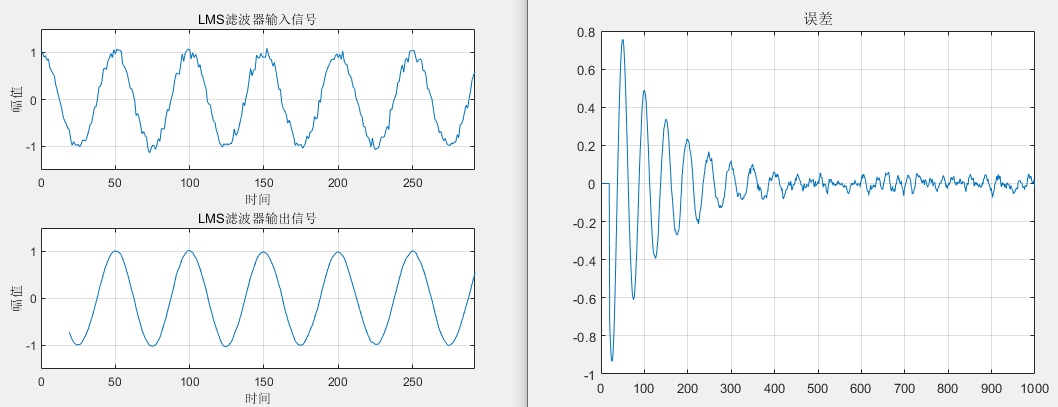这里可能有同学对期望信号会有疑惑，期望信号都是已知的了，还滤波干嘛？LMS滤波器还有什么用？

LMS滤波器的应用场景比较多，比如在机器学习中，期望确实是已知的，我们希望通过迭代训练出合适的滤波器系数；
在语音信号的线性预测中，将延时后的输入信号作为参考信号$d(n)$，即$d(n)=x(n-i)$；
在自适应回音消除中，期望信号的输入就是回声通道产生的回声。

LMS算法的优缺点：

优点：算法简单，易于实现，算法复杂度低，能够抑制旁瓣效应
缺点

收敛速率较慢，因为LMS滤波器系数更新是逐点的（每来一个新的$x(n)$和$d(n)$，滤波器系数就更新一次）,每一次采样点梯度的估计对于真实梯度会存在误差，导致滤波器系数的每次更新不会严格按照真实梯度方向更新，而是有一定的偏差
跟踪性能较差，并且随着滤波器阶数(步长参数)升高，系统的稳定性下降
LMS要求不同时刻的输入向量$x(n)$线性无关——LMS 的独立性假设。如果输入信号存在相关性，会导致前一次迭代产生的梯度噪声传播到下一次迭代，造成误差的反复传播，收敛速度变慢，跟踪性能变差。

正是由于LMS算法的缺陷，后面才有了NLMS、RLS等算法，我们会在后面的文章中一一讲到。
附：上述仿真的Python代码如下：
# This is a sample Python script.

# Press Shift+F10 to execute it or replace it with your code.
# Press Double Shift to search everywhere for classes, files, tool windows, actions, and settings.

import numpy as np
import matplotlib.pyplot as plt
import scipy.io as scio

# 信号加噪
def awgn(x, snr):
snr = 10 ** (snr / 10.0)
xpower = np.sum(np.abs(x) ** 2) / len(x)
npower = xpower / snr
if type(x) != np.complex128:
return x + np.random.randn(len(x)) * np.sqrt(npower)
else:
return x + np.random.randn(len(x)) * np.sqrt(npower / 2) + 1j * np.random.randn(len(x)) * np.sqrt(npower / 2)

def lmsFunc(xn, dn, M, mu):
itr = len(xn)
en = np.zeros((itr, 1))
W = np.zeros((M, itr))
for k in range(M, itr):
if k==20:
x = xn[k-1::-1]
else:
x = xn[k-1:k-M-1:-1]
try:
y = np.dot(W[:, k - 2], x)
print(y)
except:
pass
en[k-1] = dn[k-1] - y
W[:, k-1] = W[:, k - 2] + 2 * mu * en[k-1] * x

yn = np.ones(xn.shape) * np.nan
for k in range(M, len(xn) ):
if k == 20:
x = xn[k - 1::-1]
else:
x = xn[k - 1:k - M - 1:-1]
yn[k] = np.dot(W[:, -2], x)

return yn, W, en

if __name__ == '__main__':
fs = 1
f0 = 0.02
n = 1000
t = np.arange(n)/fs
xs = np.cos(2*np.pi*f0*t)
ws = awgn(xs, 20)
# xs = data1['xs'].flatten()
# ws = data2['ws'].flatten()

M = 20
xn = ws
dn = xs
mu = 0.001
yn, W, en = lmsFunc(xn, dn, M, mu)

plt.figure()
plt.subplot(211)
plt.plot(t, ws)
plt.subplot(212)
plt.plot(t, yn)

plt.figure()
plt.plot(en)
plt.show()

欢迎关注微信公众号：Quant_Times
欢迎大家学习我的课程：
System Generator & HLS数字信号处理教程展开全文最小均方误差 梯度下降
• 使用自适应滤波器和最小均方 (LMS) 进行有源噪声控制包含一个m文件和2个音频样本文件。matlab
• 自适应滤波器设计及Matlab实现.doc》由会员分享，可免费在线阅读全文，更多与《(终稿)自适应滤波器设计及Matlab实现.doc(OK版)》相关文档资源请在帮帮文库(www.woc88.com)数亿文档库存里搜索。1、曼滤波是线性无偏...
《自适应滤波器设计及Matlab实现.doc》由会员分享，可免费在线阅读全文，更多与《(终稿)自适应滤波器设计及Matlab实现.doc(OK版)》相关文档资源请在帮帮文库(www.woc88.com)数亿文档库存里搜索。1、曼滤波是线性无偏最小方差滤波递推滤波，它能使滤波器工作在平稳的或非平稳的环境，得到最优解。利用卡尔曼滤波理论的递推求解法导出自适应滤波器更新权矢量得不2、此为依据自动调整自己的参数，以达到最佳滤波效果。现在广为应用的自适应滤波方法主要是基于以下几种基本理论，再融合递推算法导出来的：()基于维纳滤波理论的3、出来的：()基于维纳滤波理论的方法维纳滤波是在最小均方误差准则下通过求解维纳霍夫方程来解决线性最优滤波问题的。基于维纳滤波原理，我们利用相关的瞬时值通4、同bs一旦输入信号的统计特性发生变化，应滤波器时不需要事先知道关于输入信号和噪声的统计特性的知识，它能够在自己的工作过程中逐渐估计出所需的统计特性，并5、sWidrowB和Hoff于年提出的自适应滤波理论，可使在设计自适应滤波器时不需要事先知道关于输入信号和噪声的统计特性的知识，它能够在自己的工作过程中6、是逼近的算法，使得实际估计值和理论值之间必然存在差距，也就造成在实际的应用中，往往无法得到这些统计特性的先验知识，或者统计特性是随时间变化的，因此，这7、器性能重新达到最佳。自适应滤波器自动调节参数可以通过各种不同的递推算法来实现，由于它采用的是逼近的算法，使得实际估计值和理论值之间必然存在差距，也就造8、)的基础上的噪声去除；现代滤波技术部分内容简介：了自适应滤波问题没有唯一的解。bs自适应滤波器自动调节参数可以通过各种不同的递推算法来实现，由于它采用9、逐渐估计出所需的统计特性，并以此为依据自动调整自己的参数，以达到最佳滤波效果。一旦输入信号的统计特性发生变化，它又能够跟踪这种变化，自动调整参数，使滤10、在工作过程中的逐步调整参数逼近信号的统计特性，实现最优滤波。由此，我们得到一种最常用的算法最小均方算法，简称LMS算法。()基于卡尔曼滤波理论的方法卡11、了自适应滤波问题没有唯一的解。依照各种递推算法的特点，我们把它应用于不同的场合。现在广为应用的自适应滤波方法主要是基于以下几种基本理论，再融合递推算法12、法维则是不需要知道图像的先验知识，只是根据观测数据，即可对噪声进行有效滤除。nb传统滤波技术是建立在已知有用信号和干扰噪声的统计特性(自相关函数或功率
展开全文• 本书主要介绍自适应滤波器，内容包括：自适应LMS横向滤波器，自适应格型滤波器，自适应递归滤波器，频域和子带自适应滤波器，盲自适应滤波器，神行网络非线性自适应滤波器等。
•   前一篇文章我们讲了LMS自适应滤波器，我们先回顾一下LMS算法流程： yy(n)=wT(n)x(n)e(n)=d(n)−y(n)w(n+1)=w(n)+2μe(n)x(n) yy(n)=\boldsymbol{w}^{T}(n) \boldsymbol{x}(n) \\ \boldsymbol{e}(n)=d(n)-y(n) \\...
  前一篇文章我们讲了LMS自适应滤波器，我们先回顾一下LMS算法流程：
$yy(n)=\boldsymbol{w}^{T}(n) \boldsymbol{x}(n) \\ \boldsymbol{e}(n)=d(n)-y(n) \\ \boldsymbol{w}(n+1)=\boldsymbol{w}(n)+2 \mu e(n) \boldsymbol{x}(n)$
影响LMS性能的因素，也就是最后一个公式的三个因素：

步长$u$，它是由我们事先指定
输入向量$\mathbf{x}(n)$
估计误差$e(n)$

如果$\mathbf{x}(n)$过大，那么$2ue(n)\mathbf{x}(n)$的结果中，$\mathbf{x}(n)$占的权重就太大了，而$\mathbf{x}(n)$是带噪信号，这样梯度噪声就被放大了。为了克服这个问题，可使用归一化LMS滤波器。在迭代时，对输入向量$\mathbf{x}(n)$*欧式范数（就是模值）*的平方进行归一化（Normalized LMS)。
归一化LMS滤波器是最小化干扰原理的一种表现形式，这个原理可以表述如下：

从一次迭代到下一次中，自适应滤波器的权向量应当以最小方式改变，而且受到更新的滤波器输出所施加的约束。

用$\hat{\mathbf{w}}(n)$表示第n次迭代滤波器的权向量，$\hat{\mathbf{w}}(n+1)$表示第n+1次迭代滤波器的权向量，那么NLMS设计准则可表述为约束优化问题：给定输入向量$\mathbf{x}(n)$和目标响应$d(n)$，确定更新抽头向量$\hat{\mathbf{w}}(n+1)$，以使如下增量
$\delta \hat{\mathbf{w}}(n+1)=\hat{\mathbf{w}}(n+1)-\hat{\mathbf{w}}(n)$
的欧式范数最小化，并受制于以下约束条件
$\hat{\mathbf{w}}^{\mathrm{H}}(n+1) \mathbf{x}(n)=d(n)$
使用拉格朗日乘子法来解决这个约束问题，那么代价函数为：
$J(n)=\|\delta \hat{\mathbf{w}}(n+1)\|^{2}+\operatorname{Re}\left[\lambda^{*}\left(d(n)-\hat{\mathbf{w}}^{\mathrm{H}}(n+1) \mathbf{x}(n)\right)\right]$
其中，$\lambda$为复数拉格朗日乘子，$*$表示复共轭，$Re[\cdot]$表示取实部运算，约束对代价函数的贡献是实值的；$\|\delta \hat{\mathbf{w}}(n+1)\|^{2}$表示欧式范数的平方运算，其结果也是实数。因此，代价函数$J(n)$是实值的二次函数，且可表示为：
$J(n)=(\hat{\mathbf{w}}(n+1)-\hat{\mathbf{w}}(n))^{\mathrm{H}}(\hat{\mathbf{w}}(n+1)-\hat{\mathbf{w}}(n))+\operatorname{Re}\left[\lambda^{*}\left(d(n)-\hat{\mathbf{w}}^{\mathrm{H}}(n+1) \mathbf{x}(n)\right)\right]$
采用如下步骤寻找最小的最优更新权向量：

代价函数$J(n)$对$\hat{\mathbf{w}}(n+1)$求导，可得：

$\frac{\partial J(n)}{\partial \hat{\mathbf{w}}^{\mathrm{H}}(n+1)}=2(\hat{\mathbf{w}}(n+1)-\hat{\mathbf{w}}(n))-\lambda^{*} \mathbf{x}(n)$
令其为零，即得最优解为：
$\hat{\mathbf{w}}(n+1)=\hat{\mathbf{w}}(n)+\frac{1}{2} \lambda^{*} \mathbf{x}(n)$

将上式带入约束条件，求解未知乘子$\lambda$：

\begin{aligned} d(n) &=\hat{\mathbf{w}}^{\mathrm{H}}(n+1) \mathbf{x}(n) \\ &=\left(\hat{\mathbf{w}}(n)+\frac{1}{2} \lambda^{*} \mathbf{x}(n)\right)^{\mathrm{H}} \mathbf{x}(n) \\ &=\hat{\mathbf{w}}^{\mathrm{H}}(n) \mathbf{x}(n)+\frac{1}{2} \lambda \mathbf{u}^{\mathrm{H}}(n) \mathbf{x}(n) \\ &=\hat{\mathbf{w}}^{\mathrm{H}}(n) \mathbf{x}(n)+\frac{1}{2} \lambda\|\mathbf{x}(n)\|^{2} \end{aligned}
可求得：
$\lambda=\frac{2 e(n)}{\|\mathbf{u}(n)\|^{2}}$
其中，
$e(n)=d(n)-\hat{\mathbf{w}}^{\mathrm{H}}(n) \mathbf{x}(n)$
是误差信号。

结合前两步的结果，可得：

\begin{aligned} \delta \hat{\mathbf{w}}(n+1) &=\hat{\mathbf{w}}(n+1)-\hat{\mathbf{w}}(n) \\ &=\frac{1}{\|\mathbf{x}(n)\|^{2}} \mathbf{x}(n) e^{*}(n) \end{aligned}
为了对一次迭代到下一次迭代抽头权向量的增量变化进行控制而不改变向量的方向，引入一个正的实数标度因子$u$，该增量可以写为：
\begin{aligned} \delta \hat{\mathbf{w}}(n+1) &=\hat{\mathbf{w}}(n+1)-\hat{\mathbf{w}}(n) \\ &=\frac{\widetilde{\mu}}{\|\mathbf{x}(n)\|^{2}} \mathbf{x}(n) e^{*}(n) \end{aligned}
等价的，我们可以写出：
$\hat{\mathbf{w}}(n+1)=\hat{\mathbf{w}}(n)+\frac{\mu}{\|\mathbf{x}(n)\|^{2}} \mathbf{x}(n) e^{*}(n)$
这个公式就是归一化LMS算法抽头权向量的递归公式，为什么叫归一化呢？因为公式中对输入向量$\mathbf{x}(n)$欧式范数的平方就行了归一化。
当输入向量$\mathbf{x}(n) $较小时，$\frac{\mu}{|\mathbf{x}(n)|^{2}}$的值过小，可能导致数值计算困难的情况，为了克服这个情况，将上面的表达式改为：
$\hat{\mathbf{w}}(n+1)=\hat{\mathbf{w}}(n)+\frac{\widetilde{\mu}}{\delta+\|\mathbf{x}(n)\|^{2}} \mathbf{x}(n) e^{*}(n)$
其中，$\delta>0$。
我们总结NLMS算法的步骤如下：

如果有抽头权向量$\hat{\mathbf{w}}(n)$的先验知识，则用它来做初值，否则令$\hat{\mathbf{w}}(n)$为0
计算输出：$y(n)=\boldsymbol{w}^{T}(n) \boldsymbol{x}(n)$
计算误差：${e}(n)=d(n)-y(n)$
权重更新：$\hat{\mathbf{w}}(n+1)=\hat{\mathbf{w}}(n)+\frac{\widetilde{\mu}}{\delta+\|\mathbf{x}(n)\|^{2}} \mathbf{x}(n) e^{*}(n)$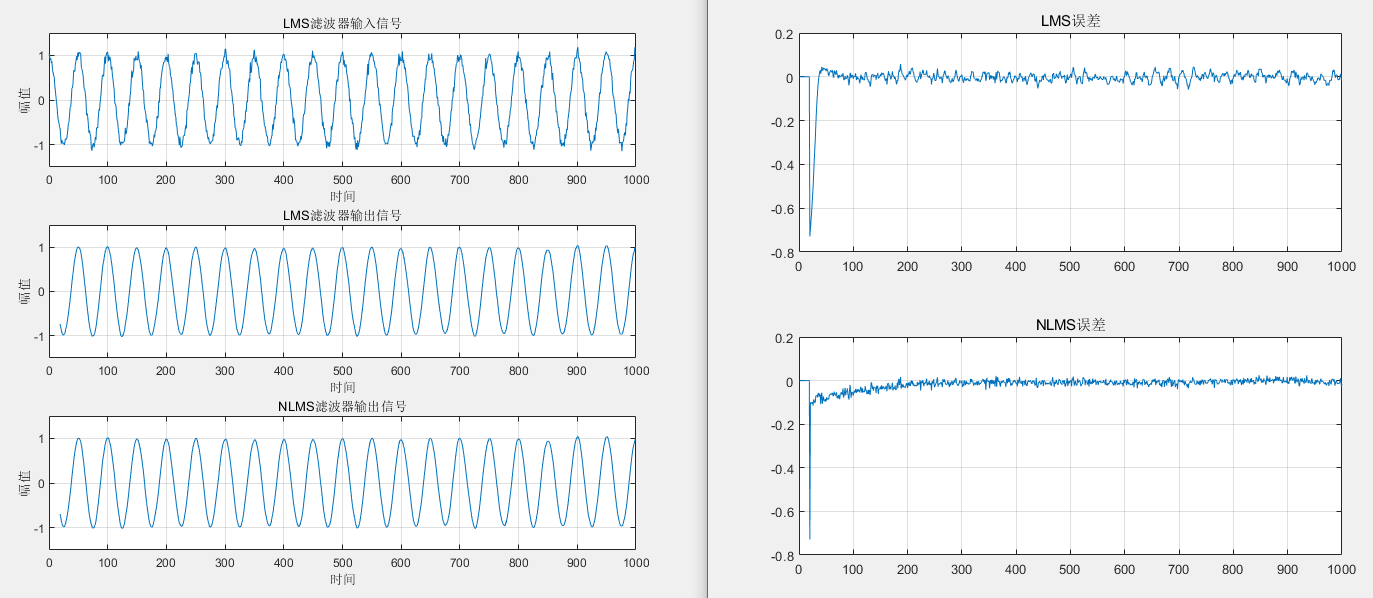% 输入参数:
%   xn   输入的信号，列向量
%   dn   所期望的响应
%   M    滤波器的阶数
%   mu   收敛因子(步长)
% 输出参数:
%   W    滤波器系数矩阵
%   en   误差序列
%   yn   滤波器输出
function [yn, W, en]=nlmsFunc(xn, dn, M, mu, delta)
itr = length(xn);
en = zeros(itr,1);
W  = zeros(M,itr);    % 每一列代表-次迭代,初始为0
% 迭代计算
for k = M:itr                  % 第k次迭代
x = xn(k:-1:k-M+1);        % 滤波器M个抽头的输入
y = W(:,k-1).' * x;        % 滤波器的输出
en(k) = dn(k) - y ;        % 第k次迭代的误差
% 滤波器权值计算的迭代式
W(:,k) = W(:,k-1) + mu*en(k)*x/(delta+x.' * x);
end

yn = inf * ones(size(xn)); % 初值为无穷大是绘图使用，无穷大处不会绘图
for k = M:length(xn)
x = xn(k:-1:k-M+1);
yn(k) = W(:,end).'* x;  % 最终输出结果
end

%% 产生测试信号
fs = 1;
f0 = 0.02;
n = 1000;
t = (0:n-1)'/fs;
xs = cos(2*pi*f0*t);
ws = awgn(xs, 20, 'measured');

M  = 20 ;   % 滤波器的阶数
xn = ws;
dn = xs;
% rho_max = max(eig(ws*ws.'));   % 输入信号相关矩阵的最大特征值
% mu = (1/rho_max) ;    % 收敛因子 0 < mu < 1/rho
mu1 = 0.01;
mu2 = 1;
delta = 1e-3;
[yn,W,en] = lmsFunc(xn,dn,M,mu1);
[yn2,W2,en2] = nlmsFunc(xn, dn, M, mu2, delta);

欢迎关注微信公众号：Quant_Times
欢迎大家学习我的课程：
System Generator & HLS数字信号处理教程展开全文自适应信号处理
• 自适应滤波器是统计信号处理的一个重要组成部分。在实际应用中，由于没有充足的信息来设计固定系数的数字滤波器，或者设计规则会在滤波器正常运行时改变，因此我们需要研究自适应滤波器。本文从自适应滤波器研究的...
• 前面学习的LMS自适应滤波器的核心思想是最速下降法，并根据当前输入信号和期望输出对代价函数的瞬时梯度进行估计。但它只使用了当前时刻的输入和期望信号，没有充分利用过去的信息。这就导致LMS自适应滤波器误差大、...
    前面学习的LMS自适应滤波器的核心思想是最速下降法，并根据当前输入信号和期望输出对代价函数的瞬时梯度进行估计。但LMS只使用了当前时刻的输入和期望信号，没有充分利用过去的信息。这就导致LMS自适应滤波器误差大、收敛慢。
一个直接的想法就是，如果能把过去的信息也都利用起来，那么梯度估计的误差就会大大减小，算法便会很快收敛。最小二乘(Least Squares，LS)算法正好就实现了这一过程，它旨在使期望输出与滤波器实际输出的残差平方和最小。
1. 最小二乘法
1.1 从维纳滤波器引出集平均
在前面介绍的维纳滤波器中提到，维纳滤波器是基于最小均方误差准则的，准则可表示为：
$\xi(n)=E[e^2(n)]=min$        　   （1）
其中e(n)是估计误差，
$e(n)=s(n)-y(n)$         　　　  　（2）
这里$\xi(n)$仅是基于当前时刻的信号。对$e^2(n)$求期望，是基于维纳滤波器要求已知估计量（即不带噪信号）的前两阶矩这一事实。因此，维纳滤波器的优化准则本质上是仅基于当前时刻信号的统计意义上的最优，即集平均。
1.2 集平均$v.s.$时间平均
集平均又称统计平均，是对一族样本进行均值估计。除了集平均外，还有一种平均方法——时间平均，它是对同一样本进行多次观察后进行均值估计。
二者区别与联系如下图所示：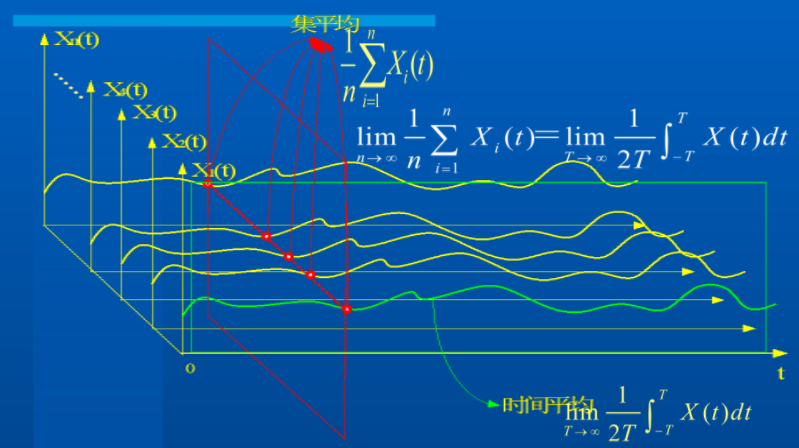集平均需要已知信号的统计特性（最起码要知道信号的一阶矩）。而时间平均则不需要知道信号的统计特性，因为它是靠多次观察来得到的。
1.3 从时间平均引出最小二乘法
和引入LMS自适应滤波器类似，重新回到前面介绍的最速下降法在维纳滤波器中的应用。
时刻$n$的估计误差再一次列出来：
$e(n)=d(n)-\bold w^T(n) \bold u(n) （3）$
最速下降法用于维纳滤波器时，是基于维纳滤波器的集平均思想，构造了基于线性最小均方误差（LMMSE）的代价函数，即需要使$E[e^2(n)]$最小。
这里，将集平均思想改为时间平均，构造新的代价函数：
$J=\sum_{n=0}^{N-1}{|e(n)|^2} （4）$
式（4）便是最小二乘法的核心思想。除了这里介绍的自适应滤波外，它还有很多其它应用，比如曲线拟合、最优化等。
2.递归最小二乘（RLS）自适应滤波器
2.1 RLS的代价函数
若将前述最小二乘法直接用于滤波，所做的将是批处理（Batch），每一次接受到新测量值的时候，需要整个模型重新计算。这样的方法需要耗费大量内存存储所有历史数据，并且计算量很大，无法进行实时滤波。
递归最小二乘法（RLS）便是为解决实时滤波问题的，其代价函数为：
$J(n)=\sum_{i=1}^{n}\lambda ^{n-i}{|e(i)|^2}+\delta \lambda^n{||\bold w(n)||^2} （5）$
说明：

式（5）的第一项表示为残差的加权平方和。$\lambda^{n-i}$是呈指数衰减的加权系数，加入权值是为了保证“遗忘”掉久远的过去数据，而更及时的跟踪最新数据，$0<λ<≈1$。事实上，经典最小二乘法假设每一时测量数据的权重相同，这样并不合理。假如一个测量值已经过去很久，那么它对计算结果的影响就应该削弱，反之影响应该加强。
式（5）的第二项是为了RLS算法推导方便加入的正则化项，随着时间的推移，$\lambda^{n}$趋向于0，正则化项的影响越来越小。$δ$是正则化系数。

2.2 计算步骤
RLS自适应滤波器的推导过程很复杂，这里直接给出推导结果，也就是实际工程应用时的计算步骤。
考虑同最速下降法用于维纳滤波同样的滤波器结构（滤波器系数长度为$M$），计算步骤如下：

算法初始化
$\hat \bold{w}(0)=\bold 0$
$\bold P(0)=\delta ^{-1}\bold I$
其中，当SNR较高时，$\delta$取小正数；当SNR较低时，$\delta$取大正数。
对每一时刻$n$，按如下步骤迭代计算
计算中间量 $\bm\pi(n)$
$\bm\pi(n)=\bold P(n-1)\bold u(n)$
计算增益向量$\bold k(n)$
$\bold k(n)=\frac{\bm\pi(n)}{\lambda+\bold u^T(n)\bm \pi(n)}$
计算先验估计误差$\bm \xi(n)$
$\xi(n)=d(n)-\bold {\hat w}^T(n-1)\bold u(n)$
更新滤波器系数$\bold w(n)$
$\bold {\hat w}(n)=\bold {\hat w}(n-1)+\bold k(n)\xi(n)$
更新逆相关矩阵$\bold P(n)$
$\bold P(n)=\lambda^{-1}\bold P(n-1)-\lambda^{-1}\bold k(n)\bold u^T(n)\bold P(n-1)$

说明如下：

$M×M$维矩阵$\bold P(n)$叫做逆相关矩阵，是输入信号相关矩阵加权和$\bm\Phi(n)$（考虑正则项）。$\bm\Phi(n)$的计算公式为：
$\bm \Phi(n)=\sum^{n}_{i=1}\lambda^{n-i}\bold u(i)\bold u^T(i)+\delta\lambda^n\bold I$
虽然$\bold P(n)$是逆相关矩阵，但因为迭代算法的存在，实际计算时并不需要矩阵求逆。
$M×1$维向量$\bold k(n)$ 叫做增益向量
$\xi(n)$是先验估计误差，计算时用到的时上一时刻的滤波器系数。不同于维纳滤波器中用到的后验估计误差。后验估计误差的计算公式为$e(n)=d(n)-\hat{\bold w}^T(n)\bold u(n)$
$\bm\pi (n)$是计算的中间量，一般$\bold k(n)$按2~3两个步骤分开计算，可减轻有限精度计算带来的问题。

2.3 RLS $v.s.$ LMS自适应滤波器
RLS自适应滤波器比LMS计算复杂，但也还好，没有矩阵求逆（LMS也没有），只有大量的乘法运算。
RLS和LMS的主要区别如下：LMS算法中的步长参数$μ$被$\bm\Phi^{-1}(n)$[即输入向量$\bold u(n)$的相关矩阵的逆]代替，它对输入信号有白化作用。
这一改进对平稳环境下RLS算法的收敛性能产生如下影响：

RLS算法的收敛速率比LMS快一个数量级。
随着迭代次数$n$趋于无线大，RLS算法的额外均方误差收敛于零。

参考文献：

https://blog.csdn.net/qinruiyan/article/details/50793114
郑宝玉 等译，自适应滤波器原理，第四版，第8章、第9章
罗鹏飞 等译，统计信号处理基础——估计理论和检测理论，第8章


展开全文最小二乘 维纳滤波器
• 自适应滤波器的参数可以调整以满足被控制对象的 未知和时变的要求 ,因此 ,在未知统计量环境中进行 信号滤波时 ,自适应滤波器较传统的固定系数滤波 器具有更高的性能 ,自适应滤波器已广泛应用于通 信 、控制 、雷达等...
• 自适应滤波器有许多种，在这里实现了基于最小均方误差自适应滤波器
• 自适应(自学习)真是个好听的词语，对懒东西最具有吸引力，因为它自己能照料自己了，不用过多的伺候了，那...自适应滤波器涉及在时间进程当中自动改变滤波器的参数(系数)来适应变化的信号特征，在过去30年中，数字信...
• 自适应滤波器设计及Matlab实现附程序代码 维纳自适应滤波器设计及 Matlab 实现摘 要本文从随机噪声的特性出发，分析了传统滤波和自适应滤波基本工作原理和性能，以及滤波技术的现状和发展前景。然后系统阐述了基本...
• 自适应滤波器原理习题答案,自适应滤波器原理,自适应滤波器原理习题答案
• 摘要：本文论述了基于TMS320F28234的自适应滤波器系统的设计方案。方案中的自适应滤波器能够在没有任何关于信号和噪声的先验知识的条件下，达到最优滤波的目的。 　根据自适应滤波的原理，主要论述和分析了易于实现......